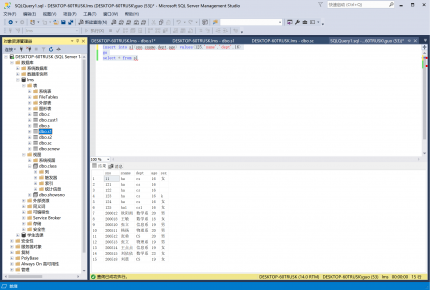# 做MSSQL实验时的一些理解c: cno课程号, cname课程名, cpno, credit学分
sc: sno, cno, score

#### 相关子查询的查询过程

```select sno,cno,score from sc as sc1
where score<(select max(score) from sc as sc2 where sc2.sno=sc1.sno)```

#### 当我们用EXISTS的时候我们在干嘛

```select count(*) from sc where exists(select * from c where c.cno=sc.cno and cname='数据库原理')
go
select count(*) from sc,c where (c.cno=sc.cno and cname='数据库原理')```

#### T-SQL拷贝表

```create table s1(
sno varchar(10) not null,sname varchar(20),dept varchar(20),age int,sex char(4))
go
insert into s1(sno,sname,dept,age,sex) select sno,sname,dept,age,sex from s```

#### 利用default约束插入数据

```insert into s1(sno,sname,dept,age) values(125,'name','dept',16)
go
select * from s1```

#### 外键约束

`alter table sc add constraint fkey foreign key(cno) references c(cno)'注释：sc表中cno的取值仅限于c表中cno的取值`
A属性的取值范围是B属性已有取值的集合。或者说A中不同元素的集合属于B中不同元素的集合。

#### SQL自定义函数（存储过程）的语法

```create proc calculate @n int '注释：@n int 是传入的参数,可以没有；create proc proc_name必须要有
as
declare @sum int,@i int '注释：声明变量，sql中变量前都加@
set @i=0 '为变量赋值
set @sum=1
while @i<@n'支持循环语句
begin
set @i=@i+1
set @sum=@sum*@i
end
print @sum
'ps：除了用set为变量赋值，也可以用select @variable= column_name from table_name where 条件```